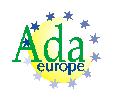# 12.5.2 Formal Scalar Types

1/2
{AI95-00442-01} A formal scalar type is one defined by any of the formal_type_definitions in this subclause. [The category class determined for a formal scalar type is the category of all discrete, signed integer, modular, floating point, ordinary fixed point, or decimal types.]
1.a/2
Proof: {AI95-00442-01} The second rule follows from the rule in 12.5 that says that the category is determined by the one given in the name of the syntax production. The effect of the rule is repeated here to give a capsule summary of what this subclause is about.
1.b/2
Ramification: {AI95-00442-01} The “category of a type” includes any classes that the type belongs to.

#### Syntax

2
formal_discrete_type_definition ::= (<>)
3
formal_signed_integer_type_definition ::= range <>
4
formal_modular_type_definition ::= mod <>
5
formal_floating_point_definition ::= digits <>
6
formal_ordinary_fixed_point_definition ::= delta <>
7
formal_decimal_fixed_point_definition ::= delta <> digits <>

#### Legality Rules

8
The actual type for a formal scalar type shall not be a nonstandard numeric type.
8.a
Reason: This restriction is necessary because nonstandard numeric types have some number of restrictions on their use, which could cause contract model problems in a generic body. Note that nonstandard numeric types can be passed to formal derived and formal private subtypes, assuming they obey all the other rules, and assuming the implementation allows it (being nonstandard means the implementation might disallow anything).
NOTES
9
12  The actual type shall be in the class of types implied by the syntactic category of the formal type definition (see 12.5, “Formal Types”). For example, the actual for a formal_modular_type_definition shall be a modular type.

#### Wording Changes from Ada 95

9.a/2
{AI95-00442-01} We change to “determines a category” as that is the new terminology (it avoids confusion, since not all interesting properties form a class).Sponsored by Ada-Europe